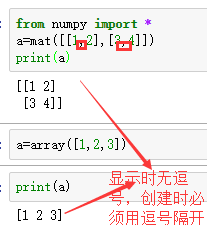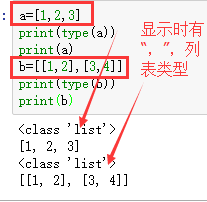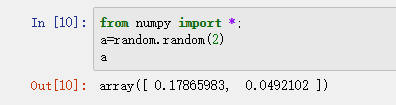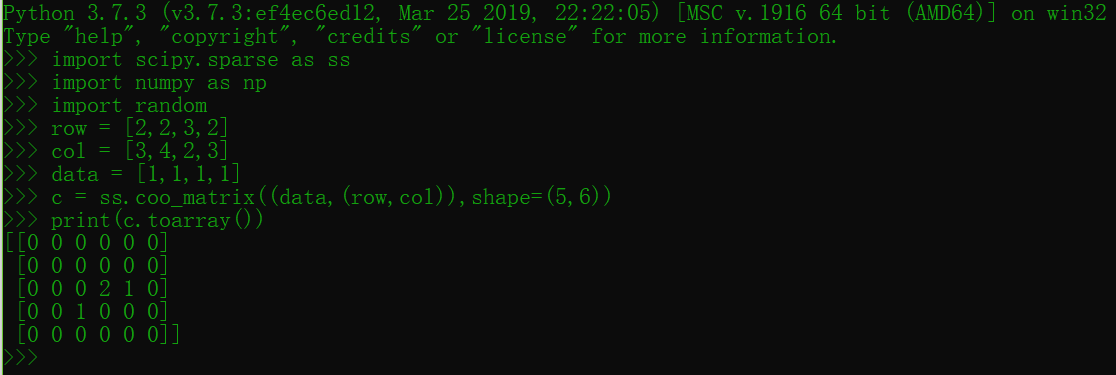• python创建矩阵There is no specific data type in Python to create a matrix, we can use list of list to create a matrix. Python中没有特定的数据类型来创建矩阵，我们可以使用list列表来创建矩阵 。 ...
python创建矩阵There is no specific data type in Python to create a matrix, we can use list of list to create a matrix.
Python中没有特定的数据类型来创建矩阵，我们可以使用list列表来创建矩阵 。
Consider the below example,
考虑下面的示例，
mat = [
[10, 20, 30],
[40, 50, 60],
[70, 80, 80]
]

It can be considered a 3x3 matrix, there are 3 rows and 3 columns in 'mat' matrix.
可以认为是3x3矩阵，“ mat”矩阵中有3行3列。
访问矩阵元素 (Accessing matrix elements)
Just like matrix in C/C++, we can access the elements in Python also.
就像C / C ++中的矩阵一样，我们也可以访问Python中的元素。
Consider the below program,
考虑下面的程序，
# Python matrix creation
mat = [
[10, 20, 30],
[40, 50, 60],
[70, 80, 80]
]

# printing the matrix
print("mat: ", mat)

# printing rows
print("mat: ", mat)
print("mat: ", mat)
print("mat: ", mat)

# printing specific elements
print("mat: ", mat)
print("mat: ", mat)
print("mat: ", mat)
print("mat: ", mat)
print("mat: ", mat)
print("mat: ", mat)
print("mat: ", mat)
print("mat: ", mat)
print("mat: ", mat)

# printing matrix using loop (matrix form)
print("Matrix is: ")
for i in range(3):
for j in range(3):
print(mat[i][j], end = " ")
print() # prints new line

Output
输出量
mat:  [[10, 20, 30], [40, 50, 60], [70, 80, 80]]
mat:  [10, 20, 30]
mat:  [40, 50, 60]
mat:  [70, 80, 80]
mat:  10
mat:  20
mat:  30
mat:  40
mat:  50
mat:  60
mat:  70
mat:  80
mat:  80
Matrix is:
10 20 30
40 50 60
70 80 80


翻译自: https://www.includehelp.com/python/program-to-create-matrix.aspxpython创建矩阵
展开全文• python编程语言
矩阵创建和矩阵运算
矩阵创建
1、from numpyimport *;
a1=array([1,2,3])
a2=mat(a1)
矩阵与方块列表的区别如下：2、data2=mat(ones((2,4)))
创建一个2*4的1矩阵，默认是浮点型的数据，如果需要时int类型，可以使用dtype=int
3、data5=mat(random.randint(2,8,size=(2,5))
产生一个2-8之间的随机整数矩阵
4、data3=mat(random.rand(2,2))
这里的random模块使用的是numpy中的random模块，random.rand(2,2)创建的是一个二维数组，需要将其转换成#matrix,并在数组中加入[0,1]之间均匀分布的随机样本
5、data4=mat(random.randn(2,2))numpy.random.randn() 官方文档中给出的用法是：numpy.random.rand(d0,d1,…dn) ,以给定的形状创建一个数组，数组元素来符合标准正态分布N(0,1)若要获得一般正态分布则可用sigma * np.random.randn(…) + mu进行表示
6、a1=random.random()
random.random()用于生成一个0到1的随机浮点数7、a1=random.rand()用于生成[0,1]之间均匀分布的随机浮点数
a=random.rand(2)

array([ 0.00760085,  0.91928957])
rand()与random()区别：
int rand(void); //返回一个随机数0 ~ pow(2, sizeof(int))-1long int random(void); //返回一个随机数0 ~ pow(2, sizeof(long int))-1

总结：rand和random的区别就是返回类型不同,int和long int(虽然在普通的32位机上效果是一样的)使用的rand或者random之前,一般使用time(0) getpid()设置随机种子.否则默认种子就是1,则每次产生的随机数都是一样的

补充：pow()用来计算以x 为底的 y 次方值，然后将结果返回。设返回值为 ret，则 ret = xy。

numpy矩阵运算
(1) 矩阵点乘：m=multiply(A,B)
(2) 矩阵乘法：m1=a*b  m2=a.dot(b)
(3) 矩阵求逆：a.I
(4) 矩阵转置：a.T

展开全文numpy
• Python矩阵创建矩阵运算 原文链接：https://blog.csdn.net/qian_fighting/article/details/79080711 矩阵创建矩阵运算 矩阵创建 1、from numpyimport *; a1=array([1,2,...
Python中矩阵创建和矩阵运算
原文链接：https://blog.csdn.net/qian_fighting/article/details/79080711

矩阵创建和矩阵运算
矩阵创建
1、from numpyimport *;
a1=array([1,2,3])
a2=mat(a1)
矩阵与方块列表的区别如下：2、data2=mat(ones((2,4)))
创建一个2*4的1矩阵，默认是浮点型的数据，如果需要时int类型，可以使用dtype=int
3、data5=mat(random.randint(2,8,size=(2,5))
产生一个2-8之间的随机整数矩阵
4、data3=mat(random.rand(2,2))
这里的random模块使用的是numpy中的random模块，random.rand(2,2)创建的是一个二维数组，需要将其转换成#matrix,并在数组中加入[0,1]之间均匀分布的随机样本
5、data4=mat(random.randn(2,2))numpy.random.randn() 官方文档中给出的用法是：numpy.random.rand(d0,d1,…dn) ,以给定的形状创建一个数组，数组元素来符合标准正态分布N(0,1)若要获得一般正态分布则可用sigma * np.random.randn(…) + mu进行表示
6、a1=random.random()
random.random()用于生成一个0到1的随机浮点数7、a1=random.rand()用于生成[0,1]之间均匀分布的随机浮点数
a=random.rand(2)

array([ 0.00760085,  0.91928957])
rand()与random()区别：
int rand(void); //返回一个随机数0 ~ pow(2, sizeof(int))-1long int random(void); //返回一个随机数0 ~ pow(2, sizeof(long int))-1

总结：rand和random的区别就是返回类型不同,int和long int(虽然在普通的32位机上效果是一样的)使用的rand或者random之前,一般使用time(0) getpid()设置随机种子.否则默认种子就是1,则每次产生的随机数都是一样的

补充：pow()用来计算以x 为底的 y 次方值，然后将结果返回。设返回值为 ret，则 ret = xy。

numpy矩阵运算
(1) 矩阵点乘：m=multiply(A,B)
(2) 矩阵乘法：m1=a*b  m2=a.dot(b)
(3) 矩阵求逆：a.I
(4) 矩阵转置：a.T


展开全文• 主要介绍了Python创建对称矩阵的方法,结合实例形式分析了Python基于numpy模块实现矩阵运算的相关操作技巧,需要的朋友可以参考下
• 1.coo_matrix >>> import scipy.sparse as ss ...稍微需要注意的一点是，用coo_matrix创建矩阵的时候，相同的行列坐标可以出现 多次 。矩阵被真正创建完成以后，相应的坐标值会 加起来 得到最终的结果。
1.coo_matrix>>> import scipy.sparse as ss
>>> import numpy as np
>>> import random
>>> row = [2,2,3,2]   #行
>>> col = [3,4,2,3]   #列
>>> data = [1,1,1,1]  #要填充的数据
>>> c = ss.coo_matrix((data,(row,col)),shape=(5,6))
>>> print(c.toarray())
[[0 0 0 0 0 0]
[0 0 0 0 0 0]
[0 0 0 2 1 0]
[0 0 1 0 0 0]
[0 0 0 0 0 0]]
>>>


稍微需要注意的一点是，用coo_matrix创建矩阵的时候，相同的行列坐标可以出现多次。矩阵被真正创建完成以后，相应的坐标值会加起来得到最终的结果。


展开全文• ## python numpy 矩阵创建

千次阅读 2018-06-04 11:22:19
1. 创建一般的多维数组import numpy as npa = np.array([1,2,3], dtype=int)type(a)a.shapea.dtype.namea.sizea.itemsizeb=np.array([1,2,3],[4,5,6], dtype=int)b.shapeb.sizeb.itemsizec = np.ones((2,3,4), dtype...
• python矩阵创建n*nFor today’s recreational coding exercise, we will look at the gravitational N-body problem. We will create a simulation of a dynamical system of particles interacting with each other...django
• python矩阵In this tutorial we will learn about Python Matrix. In our previous tutorial we learnt about Python JSON operations. 在本教程中，我们将学习Python矩阵。 在上一教程中，我们了解了Python JSON...numpy 机器学习 人工智能 tensorflow
• python提取矩阵常数In this article, we are going to create a constant matrix, where all the elements of the matrix have the same constant value. This can be done by online inbuilt function numpy.full()...numpy 机器学习 人工智能 opencv
• ## Python实现矩阵运算

千次阅读 2020-03-17 11:58:15
想用python实现一个矩阵类，它可以像matlab或者numpy中的矩阵一样进行运算。 所以我考虑了如下几点 生成一个矩阵类Matrix之后，他接收一个二维列表作为输入，然后将对应的值写到矩阵对应的位置上；如果输入为空列表...线性代数
• python 下三角矩阵A matrix can be seen in different ways and one of them is the lower triangular matrix part. Some problems in linear algebra are concerned with the lower triangular part of the matrix....numpy 机器学习 深度学习 人工智能
• 文章目录21 python numpy矩阵操作numpy 矩阵库常用矩阵操作矩阵与二维数组的转换矩阵转置 21 python numpy矩阵操作 numpy 矩阵库 numpy中包含了一个矩阵库 numpy.matlib，该模块中的函数返回的是一个矩阵，不是...
• ## python创造矩阵

万次阅读 2017-07-20 17:27:54
python的numpy创造矩阵 from numpy import mat import numpy as np data1=mat(zeros((3,3))); #创建一个3*3的零矩阵矩阵这里zeros函数的参数是一个tuple类型(3,3) data2=mat(ones((2,4))); #创建一个2...
• 如下所示： def save(data, path): f = xlwt.Workbook() # 创建工作簿 sheet1 = f.add_sheet(u'sheet1', cell_overwrite_ok=True) # 创建sheet ...以上这篇Python 保存矩阵为Excel的实现方法就是小编分
• 1：导入包numpy from numpy import * 2： 创建矩阵 A = mat([[1,2,0],[3,5,9]]) 3: 求逆矩阵 B = A.T
• 因为毕设经常要用到矩阵这样的数据结构，所以简单介绍下我知道创建矩阵的方式： （1）用链表结合append来创建，通俗的说就是链表里面放链表就构成了矩阵。 举例： list1 = [1，2 ] list2 = [3，4] matrix = ...
• python 生成对称矩阵Prerequisite: 先决条件： Defining Matrix using Numpy 使用Numpy定义矩阵 Transpose Matrix 转置矩阵 Here, we will learn how to create a symmetric matrix using a non-symmetric matrix?...机器学习 numpy tensorflow 人工智能
• python实现矩阵共轭和共轭转置 ...或使用第二种方法创建矩阵(不推荐)： >>> e = [[1, 2+3j],[3, 4+5j]] >>> e = np.matrix(a) 输出矩阵： >>> e matrix([[1.+0.j, 2.+3.j],numpy 线性代数 matlab
• python创建对角矩阵 表单是许多Web应用程序的重要组成部分，是输入和编辑基于文本的数据的最常用方法。 前端JavaScript框架（例如Angular ）通常具有自己的惯用方式来创建和验证表单，而您需要掌握这些表单才能提高...java vue javascript js
• 内容包括矩阵操作（创建矩阵、矩阵加减法）、矩阵乘法、矩阵转置（求转置矩阵）、求方阵的迹、方阵的行列式计算方法、逆矩阵/伴随矩阵、解多元一次方程。 因为要借助numpy库，如果之前没有安装过的同学，可以使用pip...numpy 线性代数
• 创建一个向量 import numpy as np #创建一个行向量 vector_row = np.array([1,2,3]) #创建一个列向量 vector_column = np.array([,,]) print(vector_row) print(vector_column) ...创建一个矩阵 import num...数据分析
• ## python求矩阵的逆

千次阅读 2020-03-06 14:51:37
文章目录准备工作 Ready to work环境 Environment模块导入 Module import代数余子式 Algebraic cofactor定义 Definition代码实现 Code余子式 Cofactor代数余子式 Algebraic cofactor伴随矩阵 Adjoint matrix定义 ...numpy 深度学习 线性代数
• 创建全X的矩阵 myzero = np.zeros([3,5]) #全0的矩阵 （2）随机矩阵 myrand = np.random.rand(3,4) (3) 单位阵 myeye = np.eye(3) (4)矩阵运算 from numpy import * 加减乘直接“+” “ -” “*” （5）矩阵元素和numpy
• ## python生成矩阵

千次阅读 2019-03-07 17:43:02
import numpy as np n=np.array(range(30)).reshape(3,10) n.reshape(3,10) 划分为三行10列
• By using numpy class we can create... 通过使用numpy类，我们可以使用两种方法创建矩阵。 Using numpy.array() 使用numpy.array() mat = numpy.array([[10,20,30],[40,50,60],[70,70,90]]) Using numpy.matrix(...numpy 深度学习 人工智能 tensorflow
• python实现矩阵乘法不使用其他库的情况下实现矩阵乘法 不使用其他库的情况下实现矩阵乘法 在不使用numpy库的情况下实现矩阵乘法，看起来很简单，但这之中也是存在坑的。比如如下代码： class mat_mul(): def mm...列表复制
• 创建矩阵用的是A=np.mat(‘1,2,3;4,5,6;7,8,9’) 调用第i行第j列时，需要使用 而不是 但是在给矩阵赋值时，就要使用 例如把一个矩阵里的值赋值给另一个矩阵： 以上内容是用np.mat创建矩阵时的操作，个人理解不...
• python numpy 矩阵编程练习 求矩阵每列的最大值 编写程序实现：用3*5的整型矩阵a接收标准输入的数据，计算数组a的每列元素的最大值，并将第i列最大值存入max相应的第i个元素。 输入格式: 标准输入的每一行表示矩阵a...numpy...

# python创建矩阵python 订阅